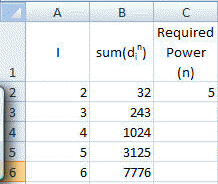﻿ Project Euler Problem 30
You are on the Home/Other Tutorials/Project Euler/Problem 30 pageShare Your

# Project Euler - Problem 30

## Problem description

Surprisingly there are only three numbers that can be written as the sum of fourth powers of their digits:

1634 = 14+ 64+ 34+ 448208 = 84+ 24+ 04+ 849474 = 94+ 44+ 74+ 44As 1 = 14is not a sum it is not included.

The sum of these numbers is 1634 + 8208 + 9474 = 19316.

Find the sum of all the numbers that can be written as the sum of fifth powers of their digits.

## Solution

I couldn't think of any slick way to solve this problem.  So, after establishing an upper limit to the range to consider, it was time for brute force.  Since it was relatively straightforward to solve in Excel I opted for it rather than VBA.

First, calculate the upper bound.  Suppose we have a 5 digit number.  The largest such number would be 99999 and the sum of each digit raised to the power of 5 would be 295245.  The same kind of analysis for a 6 digit number yields 354294.  Since each additional digit can contribute an additional 95 = 59049, the desired sum for a seven digit number will not contain more than 6 digits.  Hence, the largest number we should check is 354294.

Now, in Excel, create a list of all numbers from 2 to 354300 as below and in the adjacent column compute the sum of the digits raised to the power 5.In B2 enter the formula =SUMPRODUCT(MID(A2,ROW(INDIRECT("1:"&LEN(A2))),1)^\$C\$2) and copy it down as far down column B as required.

Now, to calculate the desired sum, use the formula =SUMPRODUCT(A2:A354300*(A2:A354300=B2:B354300))